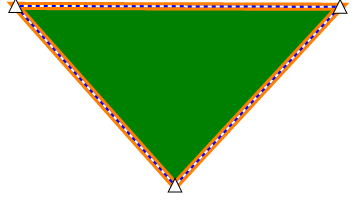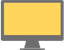Some test text!

Custom annotationskeyboard_arrow_down

# Creating custom annotations for your viewer

WebViewer allows you to create your own annotations that can be customized in several different ways. You can change the appearance and behaviors of the annotation, selection box and control handles. As an example of this we're going to walk through the steps to create a custom triangle annotation.

First let's create a basic triangle annotation "class".

``````WebViewer(
// ...
).then(function(instance) {
const { Annotations } = instance;

const TriangleAnnotation = function() {
Annotations.MarkupAnnotation.call(this);
this.Subject = 'Triangle';
};

TriangleAnnotation.prototype = new Annotations.MarkupAnnotation();

TriangleAnnotation.prototype.elementName = 'triangle';
});``````

We'll have it inherit from Annotations.MarkupAnnotation and set the elementName to triangle. The elementName is what's used for the annotation's xml element in the XFDF.

Let's override the draw function now. The draw function takes a canvas context and is called whenever the annotation should be drawn.

``````TriangleAnnotation.prototype.draw = function(ctx, pageMatrix) {
// the setStyles function is a function on markup annotations that sets up
// certain properties for us on the canvas for the annotation's stroke thickness.
this.setStyles(ctx, pageMatrix);

// first we need to translate to the annotation's x/y coordinates so that it's
// drawn in the correct location
ctx.translate(this.X, this.Y);
ctx.beginPath();
ctx.moveTo(this.Width / 2, 0);
ctx.lineTo(this.Width, this.Height);
ctx.lineTo(0, this.Height);
ctx.closePath();
ctx.fill();
ctx.stroke();
};``````

To allow a user to actually add the annotation to a document we'll need to create a tool. Our triangle just depends on two mouse points so we can inherit from the GenericAnnotationCreateTool.

``````// we also need to access the Tools namespace from the instance
const { Annotations, Tools } = instance;

// ...

const TriangleCreateTool = function(docViewer) {
// TriangleAnnotation is the constructor function for our annotation we defined previously
Tools.GenericAnnotationCreateTool.call(this, docViewer, TriangleAnnotation);
};

TriangleCreateTool.prototype = new Tools.GenericAnnotationCreateTool();``````

With our tool created we can add a button to the UI so that it can be switched to.

``````// access annotManager and docViewer objects from the instance
const { Annotations, Tools, annotManager, docViewer } = instance;

// ...

const triangleToolName = 'AnnotationCreateTriangle';

// register the annotation type so that it can be saved to XFDF files
annotManager.registerAnnotationType(TriangleAnnotation.prototype.elementName, TriangleAnnotation);

const triangleTool = new TriangleCreateTool(docViewer);
instance.registerTool({
toolName: triangleToolName,
toolObject: triangleTool,
buttonImage: '<svg xmlns="http://www.w3.org/2000/svg" width="24" height="24" viewBox="0 0 24 24" fill="currentColor">' +
'<path d="M12 7.77L18.39 18H5.61L12 7.77M12 4L2 20h20L12 4z"/>' +
'<path fill="none" d="M0 0h24v24H0V0z"/>' +
'</svg>',
buttonName: 'triangleToolButton',
tooltip: 'Triangle'
}, TriangleAnnotation);

const triangleButton = {
type: 'toolButton',
toolName: triangleToolName
};
});

// set the tool mode to our tool so that we can start using it right away
instance.setToolMode(triangleToolName);
});``````

At this point you should see a new button in the toolbar with a triangle icon, and the new triangle tool should be automatically selected. Clicking and dragging on the document should create a triangle annotation.

After creating some triangles you might notice that the selection box is a rectangle and has eight control handles. This isn't terrible but we could probably make it better by having a control handle for each corner and drawing the selection box around the edges of the annotation.

First let's change the control points so that there is one for each vertex. To do this we'll add an array of vertices on the annotation and then define a new selection model and control handles to resize the annotation.

We'll add the array to the annotation constructor:

``````const TriangleAnnotation = function() {
Annotations.MarkupAnnotation.call(this);
this.Subject = 'Triangle';
this.vertices = [];
const numVertices = 3;
for (let i = 0; i < numVertices; ++i) {
this.vertices.push({
x: 0,
y: 0
});
}
};``````

Then we'll update the draw function on the annotation to use the vertices for drawing:

``````TriangleAnnotation.prototype.draw = function(ctx, pageMatrix) {
this.setStyles(ctx, pageMatrix);

ctx.beginPath();
ctx.moveTo(this.vertices.x, this.vertices.y);
ctx.lineTo(this.vertices.x, this.vertices.y);
ctx.lineTo(this.vertices.x, this.vertices.y);
ctx.closePath();
ctx.fill();
ctx.stroke();
};``````

Then for the tool we'll override the mouseMove function to set the vertices:

``````TriangleCreateTool.prototype.mouseMove = function(e) {
// call the parent mouseMove first
Tools.GenericAnnotationCreateTool.prototype.mouseMove.call(this, e);
if (this.annotation) {
this.annotation.vertices.x = this.annotation.X + this.annotation.Width / 2;
this.annotation.vertices.y = this.annotation.Y;
this.annotation.vertices.x = this.annotation.X + this.annotation.Width;
this.annotation.vertices.y = this.annotation.Y + this.annotation.Height;
this.annotation.vertices.x = this.annotation.X;
this.annotation.vertices.y = this.annotation.Y + this.annotation.Height;

// update the annotation appearance
annotManager.redrawAnnotation(this.annotation);
}
};``````

At this point the drawing of the annotation should look the same as before, however you won't be able to move the annotation. To fix this let's create the custom selection model and control handles.

``````const TriangleControlHandle = function(annotation, index) {
this.annotation = annotation;
// set the index of this control handle so that we know which vertex it corresponds to
this.index = index;
};

TriangleControlHandle.prototype = new Annotations.ControlHandle();

// returns a rect that should represent the control handle's position and size
TriangleControlHandle.prototype.getDimensions = function(annotation, selectionBox, zoom) {
let x = annotation.vertices[this.index].x;
let y = annotation.vertices[this.index].y;
const width = Annotations.ControlHandle.handleWidth / zoom;
const height = Annotations.ControlHandle.handleHeight / zoom;

// adjust for the control handle's own width and height
x -= width * 0.5;
y -= height * 0.5;
return new Annotations.Rect(x, y, x + width, y + height);
};

// this function is called when the control handle is dragged
TriangleControlHandle.prototype.move = function(annotation, deltaX, deltaY, fromPoint, toPoint) {
annotation.vertices[this.index].x += deltaX;
annotation.vertices[this.index].y += deltaY;

// recalculate the X, Y, width and height of the annotation
let minX = Number.MAX_VALUE;
let maxX = -Number.MAX_VALUE;
let minY = Number.MAX_VALUE;
let maxY = -Number.MAX_VALUE;
for (let i = 0; i < annotation.vertices.length; ++i) {
const vertex = annotation.vertices[i];
minX = Math.min(minX, vertex.x);
maxX = Math.max(maxX, vertex.x);
minY = Math.min(minY, vertex.y);
maxY = Math.max(maxY, vertex.y);
}

const rect = new Annotations.Rect(minX, minY, maxX, maxY);
annotation.setRect(rect);
// return true if redraw is needed
return true;
};

// selection model creates the necessary control handles
const TriangleSelectionModel = function(annotation, canModify) {
Annotations.SelectionModel.call(this, annotation, canModify);
if (canModify) {
const controlHandles = this.getControlHandles();
// pass the vertex index to each control handle
controlHandles.push(new TriangleControlHandle(annotation, 0));
controlHandles.push(new TriangleControlHandle(annotation, 1));
controlHandles.push(new TriangleControlHandle(annotation, 2));
}
};

TriangleSelectionModel.prototype = new Annotations.SelectionModel();``````

Then assign the new TriangleSelectionModel as the TriangleAnnotation's selection model.

``TriangleAnnotation.prototype.selectionModel = TriangleSelectionModel;``

Now there should be a control handle for each point of the triangle and if you drag them around you'll move that vertex of the triangle! However you may notice that if you try to drag and move the annotation it won't work. To fix this let's override the resize function on the annotation.

``````TriangleAnnotation.prototype.resize = function(rect) {
// this function is only called when the annotation is dragged
// since we handle the case where the control handles move
const annotRect = this.getRect();
const deltaX = rect.x1 - annotRect.x1;
const deltaY = rect.y1 - annotRect.y1;

// shift the vertices by the amount the rect has shifted
this.vertices = this.vertices.map(function(vertex) {
vertex.x += deltaX;
vertex.y += deltaY;
return vertex;
});
this.setRect(rect);
};``````

Let's change the selection box so that it's displayed around the sides of the triangle. We'll do this by overriding the drawSelectionOutline function on the selection model.

``````TriangleSelectionModel.prototype.drawSelectionOutline = function(ctx, annotation, zoom) {
if (typeof zoom !== 'undefined') {
ctx.lineWidth = Annotations.SelectionModel.selectionOutlineThickness / zoom;
} else {
ctx.lineWidth = Annotations.SelectionModel.selectionOutlineThickness;
}

// changes the selection outline color if the user doesn't have permission to modify this annotation
if (this.canModify()) {
ctx.strokeStyle = Annotations.SelectionModel.defaultSelectionOutlineColor.toString();
} else {
ctx.strokeStyle = Annotations.SelectionModel.defaultNoPermissionSelectionOutlineColor.toString();
}

ctx.beginPath();
ctx.moveTo(annotation.vertices.x, annotation.vertices.y);
ctx.lineTo(annotation.vertices.x, annotation.vertices.y);
ctx.lineTo(annotation.vertices.x, annotation.vertices.y);
ctx.closePath();
ctx.stroke();

const dashUnit = Annotations.SelectionModel.selectionOutlineDashSize / zoom;
const sequence = [dashUnit, dashUnit];
ctx.setLineDash(sequence);
ctx.strokeStyle = 'rgb(255, 255, 255)';
ctx.stroke();
};

TriangleSelectionModel.prototype.testSelection = function(annotation, x, y, pageMatrix) {
// the canvas visibility test will only select the annotation
// if a user clicks exactly on it as opposed to the rectangular bounding box
return Annotations.SelectionAlgorithm.canvasVisibilityTest(annotation, x, y, pageMatrix);
};``````

For fun let's also override the control handle's draw function to make them look like triangles as well.

``````TriangleControlHandle.prototype.draw = function(ctx, annotation, selectionBox, zoom) {
const dim = this.getDimensions(annotation, selectionBox, zoom);
ctx.fillStyle = '#FFFFFF';
ctx.beginPath();
ctx.moveTo(dim.x1 + (dim.getWidth() / 2), dim.y1);
ctx.lineTo(dim.x1 + dim.getWidth(), dim.y1 + dim.getHeight());
ctx.lineTo(dim.x1, dim.y1 + dim.getHeight());
ctx.closePath();
ctx.stroke();
ctx.fill();
};``````

If everything went well you should have triangle annotations that look something like this:If you tried to save and load this annotation through XFDF you would notice that it isn't able to be reloaded. This is because WebViewer doesn't know that it needs to save the vertices array. To do this we can override the serialize and deserialize functions which are called when the annotation should be saved or loaded respectively.

``````TriangleAnnotation.prototype.serialize = function(element, pageMatrix) {
const el = Annotations.MarkupAnnotation.prototype.serialize.call(this, element, pageMatrix);
el.setAttribute('vertices', Annotations.XfdfUtils.serializePointArray(this.vertices, pageMatrix));
return el;
};

TriangleAnnotation.prototype.deserialize = function(element, pageMatrix) {
Annotations.MarkupAnnotation.prototype.deserialize.call(this, element, pageMatrix);
this.vertices = Annotations.XfdfUtils.deserializePointArray(element.getAttribute('vertices'), pageMatrix);
}``````

After making this change you should be able to export XFDF and import the string back

Get the answers you need: Support

close

## Free Trial

Get unlimited trial usage of PDFTron SDK to bring accurate, reliable, and fast document processing capabilities to any application or workflow.

Select a platform to get started with your free trial.

Unlimited usage. No email address required.